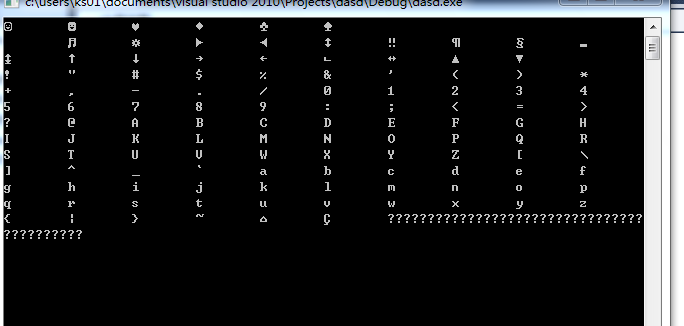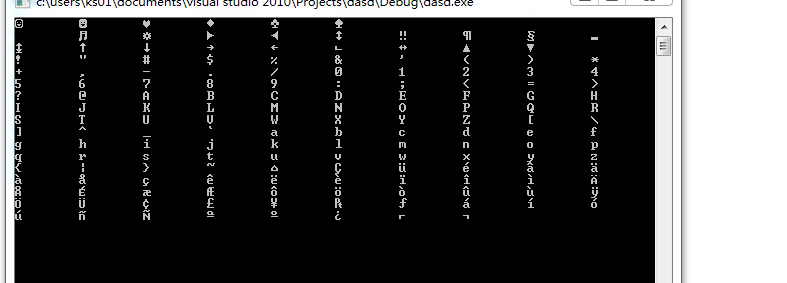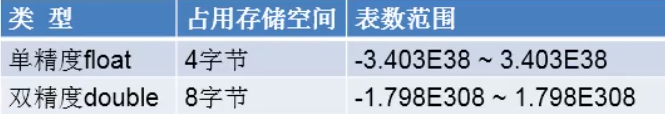• ## 浮点型变量

千次阅读 2013-09-27 23:58:24
浮点型变量是一个变量，它可以容纳一个真正的数字，如4.0，2.5，3.33，或0.1226。 有三种不同的浮点数据类型： 浮点， 双精度和长双 。 通常是一个浮动的4个字节，双8个字节，但这些都不是严格的要求，因此...

计数整数整数是伟大的，但有时我们需要存储非常大的数字，或数字带有小数部分。 浮点型变量是一个变量，它可以容纳一个真正的数字，如4.0，2.5，3.33，或0.1226。 有三种不同的浮点数据类型： 浮点， 双精度和长双 。 通常是一个浮动的4个字节，双8个字节，但这些都不是严格的要求，因此尺寸可能会发生变化。 龙双打被添加后，它的发布架构，支持更大的浮点数的语言。 但通常情况下，他们也是8个字节，相当于一个双。 总是签署浮点数据类型（可容纳正值和负值）。

下面是一些浮点数的声明：

1

2

3

float

fValue;

double

dValue;

long

double 
dValue2;

The floating part of the name floating point refers to the fact that a floating point number can have a variable number of decimal places. For example, 2.5 has 1 decimal place, whereas 0.1226 has 4 decimal places.

When we assign numbers to floating point numbers, it is convention to use at least one decimal place. This helps distinguish floating point values from integer values.

1

2

int

nValue = 5; //
5 means integer

float

fValue = 5.0; //
5.0 means floating point

How floating point variables store information is beyond the scope of this tutorial, but it is very similar to how numbers are written in scientific notation. Scientific notation is a useful shorthand for writing lengthy numbers in a concise
manner. In scientific notation, a number has two parts: the significand, and a power of 10 called an exponent. The letter ‘e’ or ‘E’ is used to separate the two parts. Thus, a number such as 5e2 is equivalent to 5 * 10^2, or 500. The number 5e-2 is equivalent
to 5 * 10^-2, or 0.05.

In fact, we can use scientific notation to assign values to floating point variables.

1

2

3

4

5

double

dValue1 = 500.0;

double

dValue2 = 5e2; //
another way to assign 500

double

dValue3 = 0.05;

double

dValue4 = 5e-2; //
another way to assign 0.05

Furthermore, if we output a number that is large enough, or has enough decimal places, it will be printed in scientific notation:

1

2

3

4

5

6

7

8

9

10

11

#include
<iostream>

int

main()

{

    using

namespace 
std;

    double

dValue = 1000000.0;

    cout
<< dValue << endl;

    dValue
= 0.00001;

    cout
<< dValue << endl;

    return

0;

}

Outputs:
1e+006
1e-005

Precision

Consider the fraction 1/3. The decimal representation of this number is 0.33333333333333… with 3′s going out to infinity. An infinite length number would require infinite memory, and we typically only have 4 or 8 bytes. Floating point numbers can only store
a certain number of digits, and the rest are lost. The precision of a floating point number is how many digits it can represent without information loss.

When outputting floating point numbers, cout has a default precision of 6 — that is, it assumes all variables are only significant to 6 digits, and hence it will truncate anything after that.

The following program shows cout truncating to 6 digits:

1

2

3

4

5

6

7

8

9

10

11

12

#include
<iostream>

int

main()

{

    using

namespace 
std;

    float

fValue;

    fValue
= 1.222222222222222f;

    cout
<< fValue << endl;

    fValue
= 111.22222222222222f;

    cout
<< fValue << endl;

    fValue
= 111111.222222222222f;

    cout
<< fValue << endl;

}

This program outputs:
1.22222
111.222
111111

Note that each of these is only 6 digits.

However, we can override the default precision that cout shows by using the setprecision() function that is defined in a header file called iomanip.

1

2

3

4

5

6

7

8

9

10

11

#include
<iostream>

#include
<iomanip> // for setprecision()

int

main()

{

    using

namespace 
std;

    cout
<< setprecision(16); //
show 16 digits

    float

fValue = 3.33333333333333333333333333333333333333f;

    cout
<< fValue << endl;

    double

dValue = 3.3333333333333333333333333333333333333;

    cout
<< dValue << endl;

Outputs:
3.333333253860474
3.333333333333334

Because we set the precision to 16 digits, each of the above numbers has 16 digits. But, as you can see, the numbers certainly aren’t precise to 16 digits!

Variables of type float typically have a precision of about 7 significant digits (which is why everything after that many digits in our answer above is junk). Variables of type double typically have a precision of about 16 significant digits. Variables of type
double are named so because they offer approximately double the precision of a float.

Now let’s consider a really big number:

1

2

3

4

5

6

7

8

9

#include
<iostream>

int

main()

{

    using

namespace 
std;

    float

fValue = 123456789.0f;

    cout
<< fValue << endl;

    return

0;

}

Output:
1.23457e+008

1.23457e+008 is 1.23457 * 10^8, which is 123457000. Note that we have lost precision here too!

Consequently, one has to be careful when using floating point numbers that require more precision than the variables can hold.

Rounding errors

One of the reasons floating point numbers can be tricky is due to non-obvious differences between binary and decimal (base 10) numbers. In normal decimal numbers, the fraction 1/3rd is the infinite decimal sequence: 0.333333333… Similarly, consider the fraction
1/10. In decimal, this is easy represented as 0.1, and we are used to thinking of 0.1 as an easily representable number. However, in binary, 0.1 is represented by the infinite sequence: 0.00011001100110011…

You can see the effects of this in the following program:

1

2

3

4

5

6

7

8

#include
<iomanip>

int

main()

{

    using

namespace 
std;

    cout
<< setprecision(17);

    double

dValue = 0.1;

    cout
<< dValue << endl;

}

This outputs:
0.10000000000000001

Not quite 0.1! This is because the double had to truncate the approximation due to it’s limited memory, which resulted in a number that is not exactly 0.1. This is called a rounding error .

Rounding errors can play havoc with math-intense programs, as mathematical operations can compound the error. In the following program, we use 9 addition operations.

1

2

3

4

5

6

7

8

9

10

#include
<iostream>

#include
<iomanip>

int

main()

{

    using

namespace 
std;

    cout
<< setprecision(17);

    double

dValue;

    dValue
= 0.1 + 0.1 + 0.1 + 0.1 + 0.1 + 0.1 + 0.1 + 0.1 + 0.1 + 0.1;

    cout
<< dValue << endl;

}

This program should output 1, but it actually outputs:
0.99999999999999989

Note that the error is no longer in the last column like in the previous example! It has propagated to the second to last column. As you continue to do mathematical operations, this error can propagate further, causing the actual number to drift farther and
farther from the number the user would expect.

Comparison of floating point numbers

One of the things that programmers like to do with numbers and variables is see whether two numbers or variables are equal to each other. C++ provides an operator called the equality operator (==) precisely for this purpose. For example, we could write a code
snippet like this:

1

2

3

4

5

int

x = 5; //
integers have no precision issues

if

(x==5)

    cout
<< "x
is 5" 
<< endl;

else

    cout
<< "x
is not 5" 
<< endl;

This program would print “x is 5″.

However, when using floating point numbers, you can get some unexpected results if the two numbers being compared are very close. Consider:

1

2

3

4

5

6

7

8

float

fValue1 = 1.345f;

float

fValue2 = 1.123f;

float

fTotal = fValue1 + fValue2; //
should be 2.468

if

(fTotal == 2.468)

    cout
<< "fTotal
is 2.468";

else

    cout
<< "fTotal
is not 2.468";

This program prints:
fTotal is not 2.468

This result is due to rounding error. fTotal is actually being stored as 2.4679999, which is not 2.468!

For the same reason, the comparison operators >, >=, <, and <= may produce the wrong result when comparing two floating point numbers that are very close.

Conclusion

To summarize, the two things you should remember about floating point numbers:

1) Floating point numbers offer limited precision. Floats typically offer about 7 significant digits worth of precision, and doubles offer about 16 significant digits. Trying to use more significant digits will result in a loss of precision. (Note: placeholder
zeros do not count as significant digits, so a number like 22,000,000,000, or 0.00000033 only counts for 2 digits).

2) Floating point numbers often have small rounding errors. Many times these go unnoticed because they are so small, and because the numbers are truncated for output before the error propagates into the part that is not truncated. Regardless, comparisons on
floating point numbers may not give the expected results when two numbers are close.

The section on relational operators has more detail on comparing floating point numbers.

展开全文• 今天来说说起浮点型变量和字符型变量 顾名思义，浮点类型变量是用来存储带有小数的实数的。 C语言中有三种不同的浮点类型，以下是对这三种不同类型的声明示例： float Amount; /单精度型/ double; /双精度型/ ...
今天来说说起浮点型变量和字符型变量
顾名思义，浮点类型变量是用来存储带有小数的实数的。
C语言中有三种不同的浮点类型，以下是对这三种不同类型的声明示例：
float Amount; /单精度型/
double; /双精度型/
long double ； /长双精度型/
浮点型都是有符号的。
单精度浮点数：
通常占用4个字节（32位）存储空间，其数值范围为-3.4E38～3.4E38，单精度浮点数最多有7位十进制有效数字，单精度浮点数的指数用“E”或“e”表示。如果某个数的有效数字位数超过7位，当把它定义为单精度变量时，超出的部分会自动四舍五入。
双精度浮点数：
通常占8 个字节（64位）内存空间，其数值范围为1.7E-308～1.7E+308。双精度完全保证的有效数字是15位，16位只是部分数值有保证。
输出 float 使用 %f 控制符，输出 double 使用 %lf 控制符，如下所示：
#include <stdio.h>
#include <stdlib.h>
int main()
{
float a=46548.101;
float b=320.302;
float c=1.23002398;
double d=123;
double e = 78.429;
printf(“a=%f \nb=%f \nc=%f \nd=%lf \ne=%lf\n”, a, b, c, d, e);
system(“pause”);
return 0;
}
运行结果：
a=46548.101563
b=320.302000
c=1.230024
d=123.000000
e=78.429000
对代码的说明：

%f 默认保留六位小数，不足六位以 0 补齐，超过六位按四舍五入截断。

将整数赋值给 float 变量时会转换为小数。

小数默认为 double 类型，加上后缀f才是float类型。

由于内存有限，小数的精度受限，所以输出 a 时只能获得一个近似数。

字符型.
字符串，它是多个字符的集合，例如 “abc123”、“123\141\142\143”；当然也可以只包含一个字符，例如 “a”、“1”、"\63"。
不过为了使用方便，我们可以用 char 类型来专门表示一个字符，例如：
char a=‘1’;
char b=’’；’;
char c=‘X’;
char d=’ ‘; // 空格也是一个字符
char e=’\63’; //也可以使用转义字符的形式
char 称为字符类型，只能用单引号’ ‘来包围，不能用双引号" “包围。而字符串只能用双引号” "包围，不能用单引号’ '包围。
输出字符使用 %c，输出字符串使用 %s。
先看下面一段代码：
#include <stdio.h>
#include <stdlib.h>
int main()
{
char a = ‘E’;
char b = 70;
int c = 71;
int d = ‘H’;
printf(“a=%c, a=%d\n”, a, a);
printf(“b=%c, b=%d\n”, b, b);
printf(“c=%c, c=%d\n”, c, c);
printf(“d=%c, d=%d\n”, d, d);
system(“pause”);
return 0;
}
输出结果：
a=E, a=69
b=F, b=70
c=G, c=71
d=H, d=72
在ASCII码表中，E、F、G、H 的值分别是 69、70、71、72。
字符和整数没有本质的区别。可以给 char 变量一个字符，也可以给它一个整数；反过来，可以给 int 变量一个整数，也可以给它一个字符。
char 变量在内存中存储的是字符对应的 ASCII 码值。如果以 %c 输出，会根据 ASCII 码表转换成对应的字符；如果以 %d 输出，那么还是整数。
int 变量在内存中存储的是整数本身，当以 %c 输出时，也会根据 ASCII 码表转换成对应的字符。
#include <stdio.h>
int main()
{
unsigned  char A=‘0’;
while(A<=170)
{
printf("%c\t",A);
A++;
}
getchar();
getchar();
return 0;
}
如该代码后面输出有问题      ascll码表127位后面是扩展的，一般是输出不出来的。
下面介绍怎莫输出后面的扩展位。右键点击边框   选择默认值    然后点击    936（）那一行   然后选择437（OE—美国），然后点击确定，在编译就会正确输出ascll扩展位了；ascll码表算上扩展位有256位，0-127是可以直接输出的，后面的全部是扩展位，直接输出不了。
这就是今天需要说的


展开全文• 定义浮点型变量 float a; 转载于:https://www.cnblogs.com/cplusplus-study/p/4517026.html

定义浮点型变量
float a;

转载于:https://www.cnblogs.com/cplusplus-study/p/4517026.html
展开全文• Java中的浮点型变量 1. 浮点型变量 在Java语言中有两种浮点型变量，分别是float和double，他们分别称为单精度浮点型和双进度浮点型。可以用下图表示： 其中的E表示为科学技术法中的10 1. 浮点型常量的表现形式 ①十...
Java中的浮点型变量
1. 浮点型变量
在Java语言中有两种浮点型变量，分别是float和double，他们分别称为单精度浮点型和双进度浮点型。可以用下图表示：其中的E表示为科学技术法中的10
1. 浮点型常量的表现形式
①十进制数形式：如：5.12 512.0f .512(其中必须要有小数点)
②科学计数法形式：如：5.12e2 512E2 100E-2
2. 两种变量之间的简单区别
**float：**单精度，尾数可以精确到7位有效数字。但是在实际生活中的很多情况下很难满足需求。
**double：**双精度，精度是float的两倍。在大多数场合中通常采用此类型。
3. 声明
**double：**double的声明方式遵循java中变量声明规则的“先声明，后引用”
double d1 = 12;

**float：**float的声明和整型中的long类似，需要在末尾加上特定的字母。在float中需要在末尾加上“f”或者“F”
float f1 = 123131114141F;



展开全文java
• 1 浮点型变量 2 Java浮点类型常量有两种表示形式 3 使用科学记数法给浮点型变量赋值 3.1 float类型赋值时需要添加后缀F/f 3.2 浮点数的比较一 3.3 浮点数的比较二 3.4 使用BigDecimal进行浮点数的比较 3.5 ...
• C/C++变量之浮点型变量 上节介绍了char型的特点。C/C++变量之表示单个字符的数据类型 本节介绍浮点型，即小数。 1、float类型 float类型（单精度浮点类型） 用来存储带小数部分的数据。 内存中占四个字节。 ...
• c浮点型变量初始值整数[rps-include post=6557] [rps-include post = 6557] As we learn variables are used to store values and variables have type. Type provides information about the value hold in the ...java python git css 数据分析
• 处理浮点型变量值交换 #include<iostream> using namespace std; int main(){ double a=123.456;double b=456.789; int*ap,*bp; ap=(int*)(&a);//把a的地址（double*）型转化成int*型，将int*的这个值...
• 浮点型变量的使用说明 浮点型表示带小数点的数值 float表示存储数组的范围比long还大 定义float类型变量时，变量要以==‘f’或‘F‘==结尾 通常定义浮点型变量，使用doublejavajava
• 浮点型变量与零值比较 不可以将浮点型变量用“＝＝”或“!=”与零值进行比较，因为浮点型变量都有精度限制，应转化为“>=”或“”形式。 如：if ( x == 0.0 ) 应写为 if ( (x>= -EPSINON) && (x 其中，EPSINON...c 浮点数比较
• Float型（单精度浮点型变量占4字节，十进制科学计数法小数点后6位有效精度和第7位的部分精度。 Double型（双精度浮点型变量占 8 字节，十进制科学计数法小数点后15位有效精度和第16位的部分精度。 浮点数的...c语言
• 1.Java中浮点型变量有两种，一种float，一种double；float占用4个字节，double占用8个字节，程序中常使用double 2.float类型的数值有一个后缀F或者f，没有后缀的浮点数值系统会默认为double类型 1.png 3....
• 无论是float型还是double型变量都存在精度的限制，所以，使用浮点型变量的一个原则就是避免将浮点型变量用“==”或“!=”与数值进行精确比较，应该借鉴误差区间的概念，转化为“>=”或“<=”形式，假设浮点型...
• 浮点型变量不能直接进行0值比较 float类型和double类型都不能保证可以精确的存储一个小数 。如何判断一个浮点型变量是否为零？ #include<stdio.h> #define E 0.00000001 int main(void) { float a = 0.0; ...c语言
• 浮点型变量包括两种：float、double double 占用8个字节，显示精度是float型的两倍，通常使用 表示范围 -1.798E308 ~ 1.798E308 float 占用四个字节，显示8位有效数字，范围 -3.403E38 ~3.403E38 范围大于...
• (1) 浮点型的表示形式有几种？分别是什么样的格式？ 浮点型的表示形式有两种 12.4 默认为double类型 123.4F 表示为float类型 也可以用以下两种形式表达浮点类型的字面常量 十进制形式:如3.14 29.34, 353.23 科学计数...
• 带小数的数据在Java中称为浮点型浮点型可分为float类型和double类型。表2-5浮点型数据类型类型占用存储空间表数范围float4字节-3.403E38~3.403E38double8字节-1.798E308~1.798E308float类型又被称作单精度类型，...
• 1、浮点型变量(float和double) 带小数的变量在Java中称为浮点型，Java的浮点型有两种：float和double。 float类型代表单精度浮点数，占4个字节、32位。double类型代表双精度浮点数，占8个字节、64位。 Java语言的...
• 带小数的数据在java中被称作浮点型浮点型可分为double和float类型。 float类型又被称为单精度类型，尾数可以精确到7位有效数字，在很多情况下，float类型的精度很难满足需求。而double这种类型的数值精确度约是...java 编程语言
• 转化为系统可认别的浮点型是问题解决的出口。 于是增加输出 System.out.println((float)element_1/lsbzsize); 显示结果： 0.6666667 即为自己需要的。   6、   找到解决问题之法： element=( ...float java
• 带小数的数据在Java中称为浮点型浮点型可分为float类型和double类型。浮点型数据类型类型占用存储空间表数范围float4字节-3.403E38~3.403E38double8字节-1.798E308~1.798E308float类型又被称作单精度类型，尾数...
• 带小数的数据在Java中称为浮点型浮点型可分为float类型和double类型。 表2-5 浮点型数据类型 类型 占用存储空间 表数范围 float 4字节 -3.403E38~3.403E38 ...
• 带小数的数据在Java中称为浮点型浮点型可分为float类型和double类型。浮点型数据类型表数范围类型占用存储空间表数范围float4字节-3.403E38~3.403E38double8字节-1.798E308~1.798E308float类型又被称作单精度类型...
• 浮点型变量：常数中带有小数点的叫做浮点型 以下用for循环写一个摄氏度和华氏度的转换的C程序 【见 http://www.linuxidc.com/Linux/2013-08/88513.htm】 [root@www.linuxidc.com]# cat forxh.c #include &...
• 当整型变量赋值给浮点型变量的时候，对于两种不同的存储形式，计算机是怎么完成数据的复制？ 首先，编一个简单的C程序，通过编译生成的汇编文件看一下计算机是怎么处理的。 /*文件名:1.c*/ #include <stdio.h>...linux...